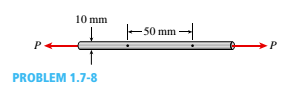# A tensile test is performed on a brass specimen 10 mm in diameter using a gage length of 50 mm (see figure). When the tensile load P reaches a value of 20 kN, the distance between the gage marks has increased by 0.122 mm. (a) What is the modulus of elasticity E of the brass? (b) H the diameter decreases by 0.00830 mm, what is poison’s ratio?### Mechanics of Materials (MindTap Co...

9th Edition
Barry J. Goodno + 1 other
Publisher: Cengage Learning
ISBN: 9781337093347### Mechanics of Materials (MindTap Co...

9th Edition
Barry J. Goodno + 1 other
Publisher: Cengage Learning
ISBN: 9781337093347
Chapter 1, Problem 1.7.8P
Textbook Problem
517 views

## A tensile test is performed on a brass specimen 10 mm in diameter using a gage length of 50 mm (see figure). When the tensile load P reaches a value of 20 kN, the distance between the gage marks has increased by 0.122 mm. (a) What is the modulus of elasticity E of the brass? (b) H the diameter decreases by 0.00830 mm, what is poison’s ratio?(a)

To determine

The modulus of elasticity E of the brass.

### Explanation of Solution

Given Information:

A tensile test is performed on a brass specimen as shown in the figure below:

Diameter of the bar d=10mm,

Gauge Length L=50mm.

Force applied P=20 KN

δl=0.122 mm

Axial Strain in the barε=δlL=0.12250=2.44×103

Axial Stress in the bar σ=Pπ4d2=20000π4(10)2=254

(b)

To determine

The Poisson’s ratio, if the diameter decreases by 0.00830 mm.

### Still sussing out bartleby?

Check out a sample textbook solution.

See a sample solution

#### The Solution to Your Study Problems

Bartleby provides explanations to thousands of textbook problems written by our experts, many with advanced degrees!

Get Started

Find more solutions based on key concepts
What is an assistive technology system?

Principles of Information Systems (MindTap Course List)

Solve Problem 20.18 using Excel.

Engineering Fundamentals: An Introduction to Engineering (MindTap Course List)

Why do app developers opt for web delivery? (161)

Enhanced Discovering Computers 2017 (Shelly Cashman Series) (MindTap Course List)

Can all electrodes be used with a leading angle? Why or why not?

Welding: Principles and Applications (MindTap Course List)

If your motherboard supports ECC DDR3 memory, can you substitute non-ECC DDR3 memory?

A+ Guide to Hardware (Standalone Book) (MindTap Course List)